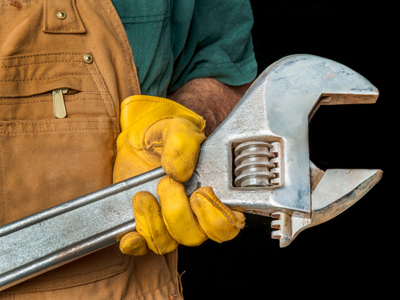Moments (turning forces) depend on force and distance from the pivot.

# Moments 01

## Questions and answers about turning forces. Can you give an example of a tool that uses moments?

### Scroll down to play the quiz

This KS3 Science quiz will test you on moments. You use moments every day of your life - opening a door, bending your leg at the knee, turning a key in a lock and so on. Forces are used to turn objects about a pivot (fixed point), the force creates a moment (a turning force). A good example is loosening or tightening a nut and bolt. Pushing or pulling the handle of a spanner connected to the nut or bolt produces a moment. The size of the moment is affected by two things - the size of the pushing or pulling force and how far from the pivot point the force is applied. If the nut is very tight, the person using the spanner must either increase the force they are using or use a longer spanner.

A moment can be calculated very easily, it is simply the size of the force multiplied by the distance that the force is from the pivot. In SI units, force is measured in newtons and distance is measure in metres so the units of a moment are newton metres. If you double the distance of a force, the moment will be doubled and vice-versa. If you halve the distance of the force, the moment will be halved.

1.
Moments (turning forces) depend on .......
force and distance from the pivot
force and mass
force and weight
mass and distance from the pivot
The unit for a moment is Nm - newton metre
2.
The moment will be increased .......
if the force and distance from the pivot are decreased
if the force and distance from the pivot are increased
if the force is decreased and distance is increased
if the force is increased and distance is decreased
Moment = force x distance
3.
Jane weighs 400 N. She sits on a see-saw 1.5 m from the pivot (centre). Calculate the moment caused by Jane.
200 Nm
400 Nm
600 Nm
800 Nm
Moment = force x distance = 400 x 1.5 = 600 Nm
4.
Amir weighs 500 N. He sits on a see-saw 200 cm from the pivot (centre). Calculate the moment caused by Amir.
200 Nm
700 Nm
1,000 Nm
10,000 Nm
Distance = 200 cm = 2 m, so the moment = 500 x 2 = 1,000 Nm
5.
Two children weigh 300 N and 400 N, they sit 2 m and 3 m away from the pivot (centre) on the same side of the see-saw. What is their total moment?
1,700 Nm
1,800 Nm
2,200 Nm
3,500 Nm
Moment = (300 x 2) + (400 x 3) = 1,800 Nm
6.
Two children are sitting on the same side of a see-saw. Their total moment is 1800 Nm. A friend joins the two children in the play area and sits on the other end of the see-saw. He weighs 600N. How far from the pivot must he sit to balance the see-saw?
2m
2.5m
3m
5m
Moment = force x distance, 1,800 Nm = 600 x ? = 3 m
7.
A spanner is 20 cm long. A force of 10 N is applied to undo a bolt. What is the moment (turning force)?
2 Nm
20 Nm
200 Nm
2,000 Nm
Distance = 20 cm = 0. 2 m moment = 10 x 0. 2 = 2 Nm. Did you remember to change cm to m?
8.
A 40 cm long spanner is used to produce the same turning force as a 20 cm long spanner. What force would be needed for the 40 cm long spanner compared to the force used on the 20 cm long spanner?
Double the force
Quarter of the force
Half the force
The same force
When you double the distance from a pivot point, you only need half of the force to create the same moment
9.
A moment of 50 Nm is needed to open a jar using a pair of pliers designed to make opening jars easier. If the pliers are 10 cm long. What force is needed?
5 N
50 N
100 N
500 N
10 cm = 0.1 m, 50 = force x 0.1, force = 50/0.1 = 500 N
10.
A moment of 80 Nm is needed to undo a wheel nut. The spanner is 20 cm long. What force is needed?
4 N
80 N
400 N
800 N
20 cm = 0.2 m, 80 = force x 0.2, force = 80/0.2 = 400 N